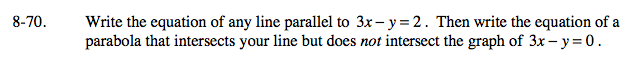Home > A2C > Chapter 8 > Lesson 8.1.4 > Problem8-70

8-70.

Write the equation of any line parallel to 3xy = 2. Then write the equation of a parabola that intersects your line but does not intersect the graph of 3xy = 0. Homework Help ✎Start by converting 3xy = 2 to slope-intercept form.

Any line with the same slope will be parallel.
Any downward-opening parabola with a vertex below the line 3xy = 0 and above 3xy = 2 will work.
One possible parabola is y = −x2 − 2.